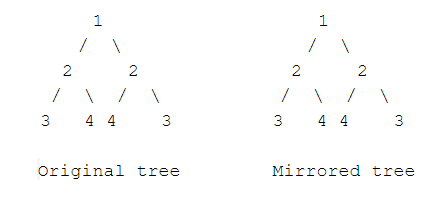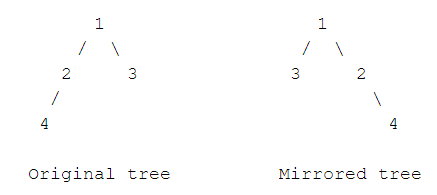2

# Tree Symmetricity

Difficulty: EASY
Avg. time to solve
20 min
Success Rate
82%

Problem Statement
Suggest Edit

#### Symmetric tree is a binary tree, whose mirror image is exactly the same as the original tree.

##### For Example:##### Input format:
``````The only line of input contains the binary tree node elements in the level order form. The values of nodes are separated by a single space in a single line. In case a node is null, we take -1 in its place.

For example, the input for the tree depicted in the below image would be :
````````````1
2 3
4 -1 5 6
-1 7 -1 -1 -1 -1
-1 -1
``````

#### Explanation :

``````Level 1 :
The root node of the tree is 1

Level 2 :
Left child of 1 = 2
Right child of 1 = 3

Level 3 :
Left child of 2 = 4
Right child of 2 = null (-1)
Left child of 3 = 5
Right child of 3 = 6

Level 4 :
Left child of 4 = null (-1)
Right child of 4 = 7
Left child of 5 = null (-1)
Right child of 5 = null (-1)
Left child of 6 = null (-1)
Right child of 6 = null (-1)

Level 5 :
Left child of 7 = null (-1)
Right child of 7 = null (-1)

The first not-null node (of the previous level) is treated as the parent of the first two nodes of the current level. The second not-null node (of the previous level) is treated as the parent node for the next two nodes of the current level and so on.

The input ends when all nodes at the last level are null (-1).
``````
##### Note :
``````The above format was just to provide clarity on how the input is formed for a given tree.

The sequence will be put together in a single line separated by a single space. Hence, for the above-depicted tree, the input will be given as:

1 2 3 4 -1 5 6 -1 7 -1 -1 -1 -1 -1 -1
``````
##### Output format:
``````The output consists of a single line containing "Symmetric" if the given tree is symmetric, else "Asymmetric".
``````
##### Note:
``````You are not required to print the expected output; it has already been taken care of, Just implement the function.
``````
##### Constraints:
``````0 <= N <= 10^5
1 <= Data <= 10^5

Where 'N' denotes the number of nodes in the given binary tree and 'Data' denotes the node value.

Time limit: 1sec
``````
##### Sample Input 1:
``````1 2 2 3 4 4 3 -1 -1 -1 -1 -1 -1 -1 -1
``````
##### Sample Output 1:
``````Symmetric
``````
##### Explanation For Sample 1:
``````This is a symmetric tree:
``````##### Sample Input 2:
``````1 2 3 4 -1 -1 -1 -1 -1
``````
##### Sample Output 2:
``````Asymmetric
``````
##### Explanation For Sample 2:
``````This is an asymmetric tree:
``````Console